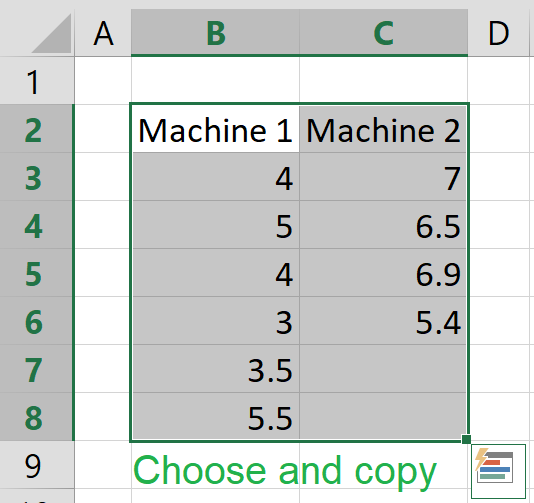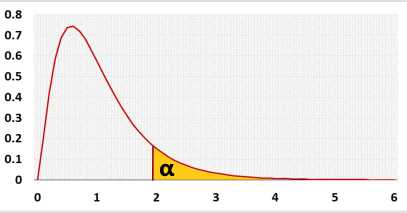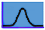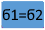# One Way ANOVA Calculator

## Analysis Of Variance, Tukey HSD test

Enter raw data directly
Enter raw data from excel

## Enter sample data directly

Header: You may change groups' name to the real names.
Data: When entering data, press Enter or , (comma) after each value.
 Groups: Group1 Group2 Group3 Data: Skewness: Excess kurtosis: Normality Outliers Mean S
Empty cells or non-numeric cells will be ignored

## Enter sample data from excel

You may copy the data from Excel, Google sheets or any tool that separate the data with Tab and Line Feed. Copy the data, one block of consecutive columns includes the header, and paste. Click to see example:Empty cells or non-numeric cells will be ignored

tresults
R code.

## Information

Target: To check if the difference between the averages of two or more groups is significant, using sample data
ANOVA is usually used when there are at least three groups since for two groups, the two-tailed pooled variance t-test and the right-tailed ANOVA test have the same result.

When performing ANOVA test, we try to determine if the difference between the averages reflects a real difference between the groups, or is due to the random noise inside each group.
The F statistic represents the ratio of the variance between the groups and the variance inside the groups. Unlike many other statistic tests, the smaller the F statistic the more likely the averages are equal.

Example: Compare four fertilizers used in four fields
H0: The average weight of crops per square meter is equal in all fields.
H1: At least one field yields different average per square meter.

Right-tailed F test, for ANOVA test you can use only the right tail. Why?
Hypotheses
H0: μ1 = .. = μk
H1: not(μ1 = .. = μk)
Test statisticF distribution## AssumptionsIndependent samplesNormal distribution of the analyzed populationEqual standard deviation, σ1=σ2=...=σkThe assumption is more important when the groups' sizes not similar.

## Required Sample DataSample data from all compared groups

## Parameters

k - Number of groups.
ni - Sample side of group i.
n - Overall sample side, includes all the groups (Σni, i=1 to k).
xi - Average of group i.
x - Overall average (Σxi,j / n, i=1 to k, j=1 to ni).
Si - Standard deviation of group i.

## Results calculations

SourceDegrees of FreedomSum of SquaresMean SquareF statisticp-value
Groups
(between groups)
k - 1MSG = SSG / (k - 1)F = MSG / MSEP(x > F)
Error
(within groups)
n - kMSE = SSE / (n - k)
Totaln - 1SS(total) = SSG + SSESample Variance = SS(total) / (n - 1)

## R Code

The following R code should produce the same results